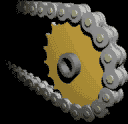﻿Bicycle chain

# The bicycle chain as a model for an electric current

Introduction
The phraseology of many students shows often a false concept about electric current.
Example l) "the flow starts at the positive pole and ends at the negative one."
Example 2) "At first the power passes the light and after it the switch."
Example 3: "There is current on."
To remove these wrong concepts — and to improve understanding immediately - the next model has been designed.## The bicycle chain

A rotating bicycle chain is a very suitable model for an electric current.
The chain can be powered either by bicycle pedals or by an electric motor.

In each point of the whole circuit a current is present simultaneously:
anywhere in the circle the chain links move in the same way.
Each link presents the same amount of electric charge, a number of coulombs.

The simulated voltage source
A portion of the chain is covered by a panel which is in the form of a battery.
The chain goes to one pole and comes out the other one.

Alternating current (AC), direct current (DC) and pulsating DC
• By moving the bicycle pedals back and forth one simulates an alternating current.
• By moving the bicycle pedals with constant speed the illusion is shown of a direct current.
• Moving with variable speed and always moving in the same direction an alternating current is shown.
This one is less known. (Think of the pulsating blood flow as a result of the heartbeat.)
Amperage
An electric current is a number of ampere.
This is a number of coulombs per second.
When the teacher says each link represents a certain amount of charge, a number of coulombs.
he can explain the electric current, the amperage, very well.
He can (let) count how many links pass each minute at any place.
Then the number of links per second shows the model of an electric current.
Counting is somewhat easier by giving the links a color.
Optional is the possibility to apply a mechanical counter.
The counting student or the mechanical counter is a model of an ammeter.

Background knowledge
The conduction electrons in a metal moving at a speed that is an order of magnitude of 100 km/s.
The direction is changing frequently by collisions with the vibrating metal ions
making them not very fast averagely. When a wire conducts a current the 'drift velocity' is only mm/s.
Therefore it is justified to move the chain very slowly. That makes counting the links a lot easier.

The current rule
Observance: the intensity of the current is the same at every point.
That is very controllable. Invite three students counting the number of links simultaneously.
They will, if they do not make mistakes, reach the same amount.
If the speed of the chain would fluctuate, it has no result on the three countings.
The three countings of the links can be done as well mechanical. That way is clearly preferred.

The result of an electrical resistance
The electrical resistance is simulated using a mechanical friction brake.
Clearly illustrated by the bicycle chain model that braking is a local happening
while the impact on the speed takes place on all links of the chain,
therefore on the amperage in the entire circuit.

Heat dissipation
By applying a thermo-meter in the brake, so the resistance,
one can determine the local rise of temperature.

Restriction
With this model branching currents cannot be demonstrated.

 Physics Science